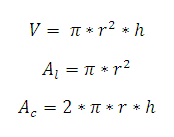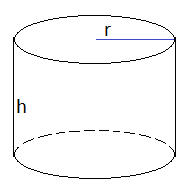# Right Circular Cylinder Calculator

This CalcTown calculator calculates the volume, lateral surface area and curved surface area of right circular cylinder.

#### Result

m3
m2
m2Where,

π = 3.14 (constant)

r = radius of the cylinder

h = height of the cylinder

V = volume of the cylinder

At = top surface area of the cylinder

Ac = curved / lateral surface area of the cylinder